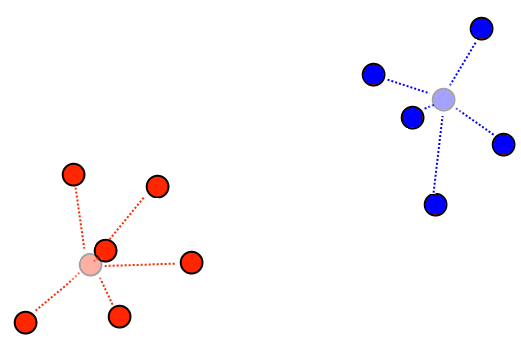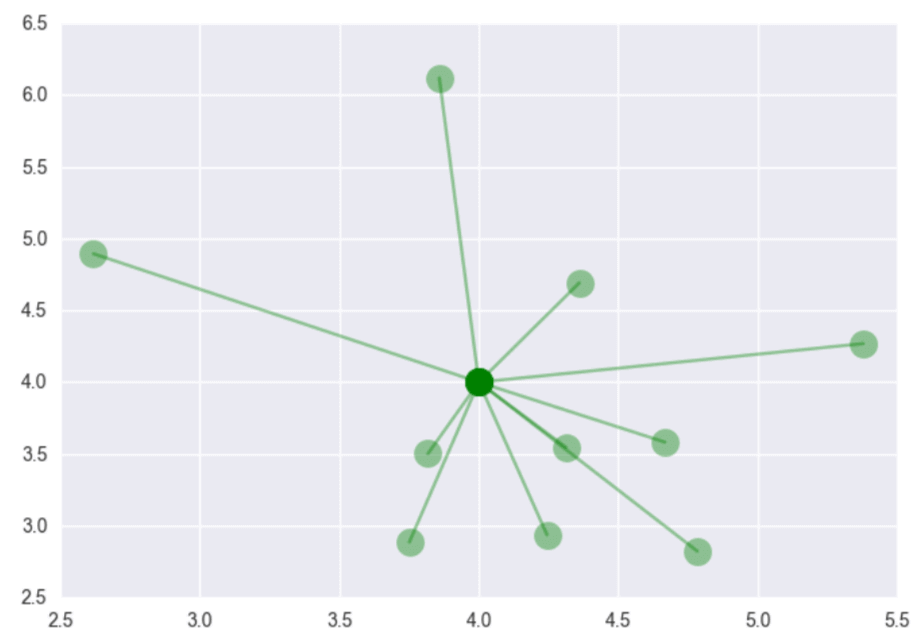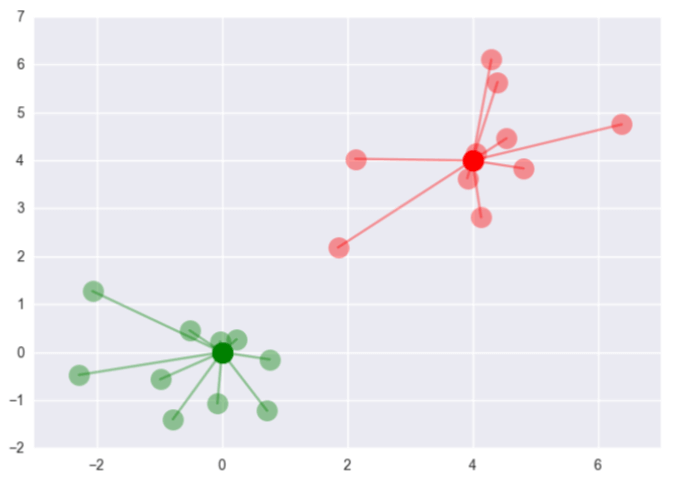#### 可视化K Means的时候怎么把聚类的中心点和样本点连起来？

统计/机器学习 无监督学习 数据可视化 Python    浏览次数：5012        分享

0

K Means每个聚类都有个中心点，有没有什么方法可以把中心点和归属它的样本点连起来？ggg818   2017-12-07 00:27

##### 1个回答
7

import matplotlib.pyplot as plt; plt.style.use('ggplot')
a = np.random.normal(4, 1, 10)
b = np.random.normal(4, 1, 10)
center = [4, 4]
for point in zip(a, b):
plt.plot([center, point], [center, point], marker='o', markersize=15, color='g', alpha=0.4)
plt.show()a = np.random.normal(0, 1, 10)
b = np.random.normal(0, 1, 10)
center = [0, 0]
for point in zip(a, b):
plt.plot([center, point], [center, point], marker='o', markersize=15, color='g', alpha=0.4)
a = np.random.normal(4, 1, 10)
b = np.random.normal(4, 1, 10)

center = [4, 4]
for point in zip(a, b):
plt.plot([center, point], [center, point], marker='o', markersize=15, color='r', alpha=0.4)
plt.show()SofaSofa数据科学社区DS面试题库 DS面经Lydia   2017-12-07 08:51相关主题